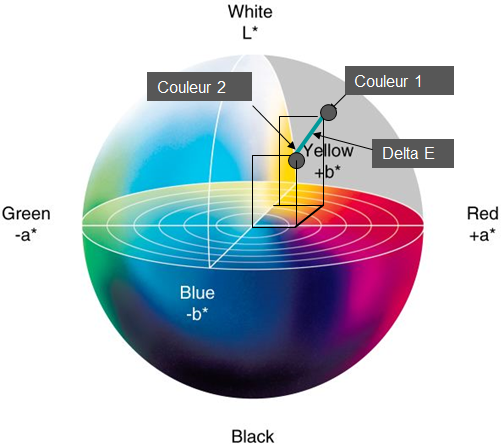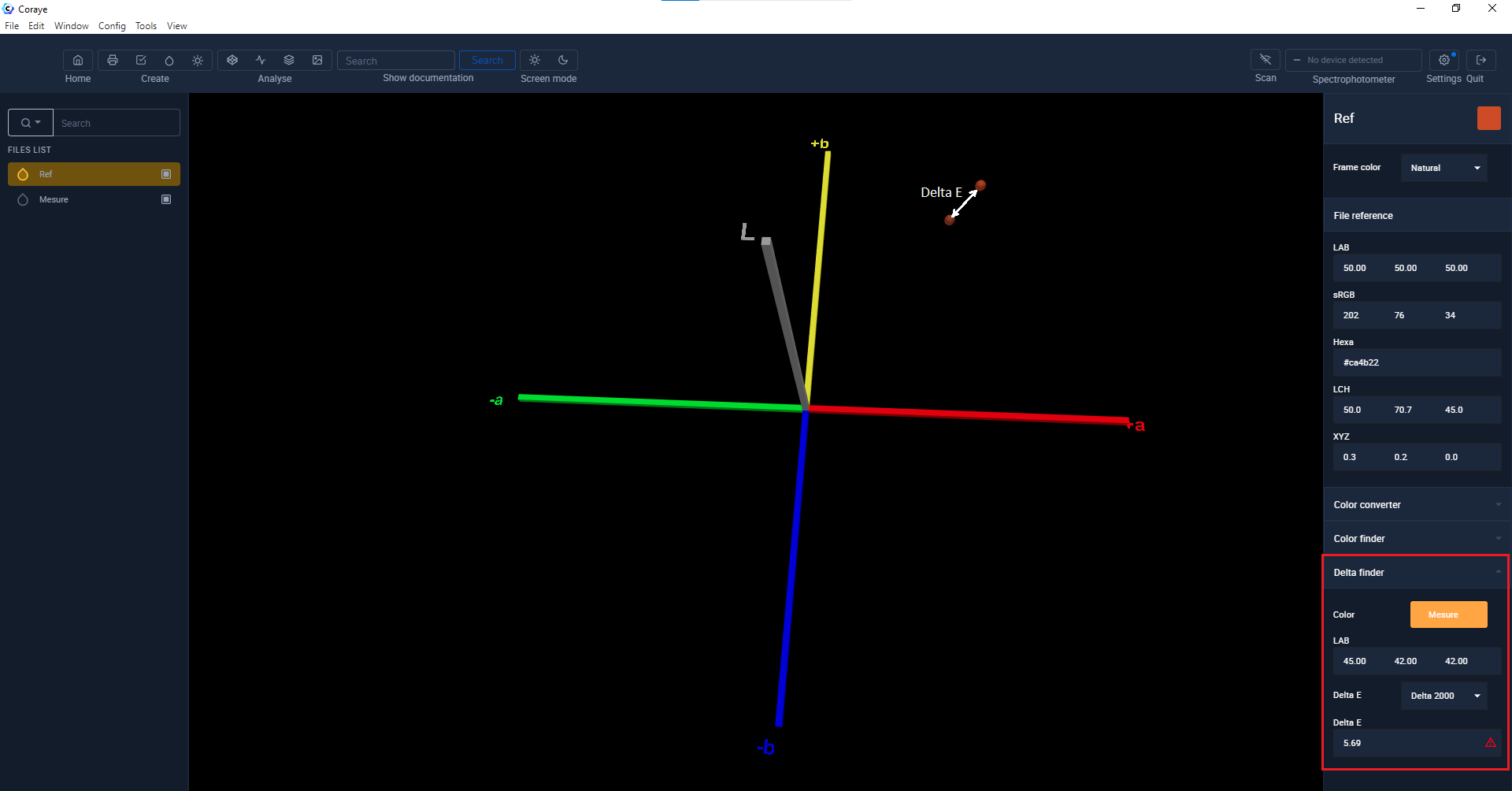# The Delta E

##### The Delta E quantifies the difference in color between two samples

This value is the difference between two colors designated as two points in the Lab color space.
The closer the Delta E value tends to 0, the greater the color accuracy.

##### Using the Delta E allows you to set up quality control procedures, compare a color with a reference color chart, or simply check if a color is reproducible on a printer.

If the Delta E is equal to 0, it means that the color 1 and the color 2 are identical.
If the Delta E is greater than 2, the human eye will be able to discern a difference between the two colors.##### ∆E is the initial of the German word "Empfindung" meaning: "Perceived difference"

The original formula for calculating the color difference ( Delta E76 ) was created in 1976 by the  International Commission on Illumination (CIE)

Color 1: Lab value: L1, a1, b1
Color 2: Lab value: L2, a2, b2We recognize the  Euclidean distance formula .

In 1994, a second version of the calculation mode ( Delta E94 ) was developed to apply different coefficients depending on the materials measured.

In 2000, a new version of the calculation mode ( Delta E00 ) made it possible to obtain a value of the delta E more consistent with the perception of the human eye.
Starting from the principle that the eye is more sensitive to color differences when the colors are close to gray (L axis) and that it is less sensitive when it compares saturated colors (example of lemon yellow) , the calculation of the Delta E2000, takes into account the color zones in its calculation mode.

To find out more about the different Delta E formulas:

http://www.brucelindbloom.com/Math.html

#### Let's compare two colors with Coraye

In the example below we have displayed a reference color and a measured color to visualize the difference between these two colors.
The value of Delta E is also displayed in the lower right corner of the window.